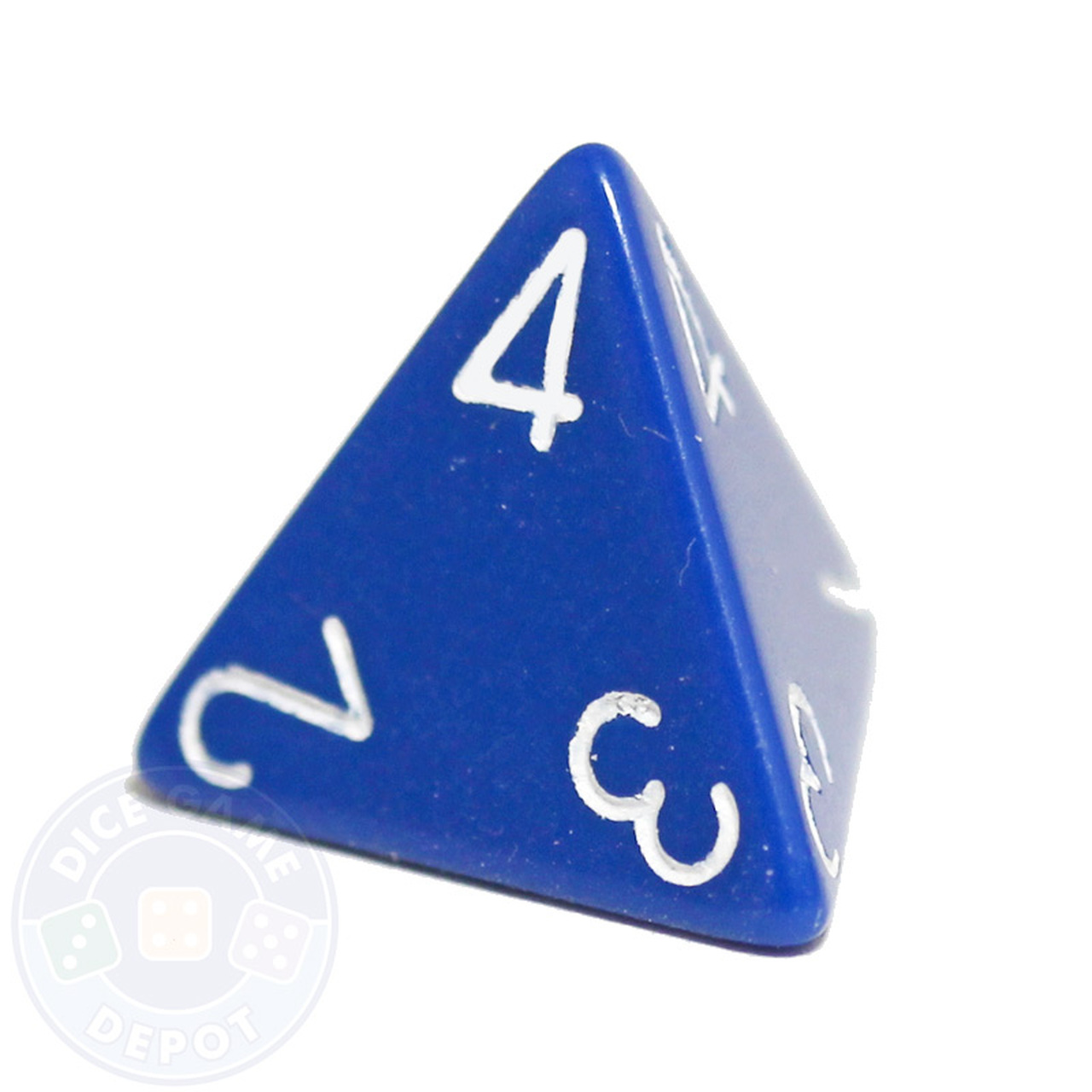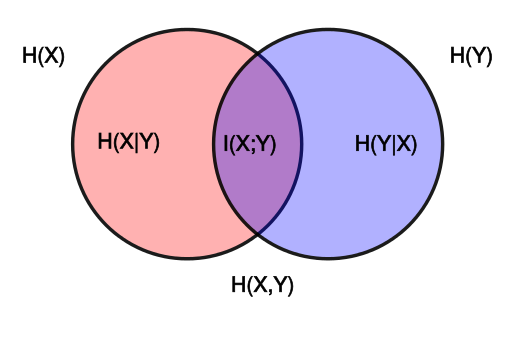# Cross entropy, joint entropy, conditional entropy and relative entropy

Marton Trencseni - Sat 09 October 2021 - Math

## Introduction

In the previous article I discussed entropy. As a follow-up, I revisit more advanced variations of entropy and related concepts:

1. Joint entropy
2. Conditional entropy
3. Cross entropy
4. Relative entropy (also known as Kullback–Leibler divergence)
5. Mutual information (also known as Information gain)

Everything I cover here is elementary information theory, mostly found in the first chapter of the classic Cover, Thomas: Elements of Information Theory or the wikipedia pages linked above.

## Entropy

Entropy is the amount of uncertainty of a random variable, expressed in bits. Imagine Alice has a random variable and she needs to communicate the outcome over a digital binary channel to Bob. What is a good encoding to minimizes the average amount of bits she sends? In the previous article I discussed the case of a fair coin, so let's make it a bit more complicated here, and use a 4-sided dice, ie. a tetrahedron. Let's say it's a fair tetrahedron, so each side comes up with $p = \frac{1}{4}$ probability.There are 4 outcomes, so she needs to use 2 bits:

1 -> 00
2 -> 01
3 -> 10
4 -> 11


Since all outcomes are equally likely, there is no reason for her to deviate from this trivial encoding. So, on average she will use 2 bits per message.

What if one of the sides is more likely to come up? Suppose the distribution is $p = [\frac{1}{2}, \frac{1}{6}, \frac{1}{6}, \frac{1}{6}]$. In this case, it makes sense to use less bits for the first side, since it will come up more, so she sends less bits on average. Eg. she can try:

1 -> 0
2 -> 10
3 -> 110
4 -> 111


With this encoding, on average she's sending $[\frac{1}{2}, \frac{1}{6}, \frac{1}{6}, \frac{1}{6}] \times [1, 2, 3, 3] = \frac{11}{6} = 1.83$ bits per message.

The formula for entropy is $H(X) = - \sum_i p(x_i) log[p(x_i)]$. For the fair tetrahedron, $H(X)=2$, which means that the trivial encoding is optimal. For the biased tetrahedron above, $H(X)=1.79$ bits, which is less than the encoding above. This means that there are better encodings, we can still save about 0.04 bits per message. To do this, we need to do block encoding, encode several messages, like:

11 -> 0
12 -> ...
14 -> ...
14 -> ...
21 -> ...
...


By creating larger and larger block encodings, we can arbitrarily approach the theoretical limit of $H(X)$, but we can never do better than that.

The formula $H(X) = - \sum_i p(x_i) log[p(x_i)]$ for entropy can be made intuitive: the number of bits we should use to encode an outcome with probability $p(x_i)$ is of length $- log[p(x_i)]$ bits. Eg. for $p(x_i) = \frac{1}{2}$ this is 1 bit, for $p(x_i)= \frac{1}{4}$ this is 2 bits. Considering the examples above, this is intuitive. And the $p(x_i)$ multiplier is just how often this outcome will occur. So it makes sense that this is the average number of bits sent per message.

Also note the entropy of a random variable that has just one outcomes is 0, we don't need any bits if we already know what the outcome is going to be.

## Joint entropy

Just as there is probability $p(x)$, $p(y)$ and joint probability $p(x, y)$, we can define entropy $H(X)$ and joint entropy $H(X, Y)$.

$H(X, Y) = - \sum_{ij} p(x_i, y_j)log[p(x_i, y_j)]$

If $X$ and $Y$ are independent, then $H(X, Y) = H(X) + H(Y)$. This is easy to see, because then $p(x, y) = p(x) p(y)$, so:

$H(X, Y) = - \sum_{ij} p(x_i, y_j)log[p(x_i, y_j)] = - \sum_{ij} p(x_i)p(y_j)log[p(x_i)p(y_j)]$

Now we use $log(ab)=log(a)+log(b)$:

$H(X, Y) = - \sum_{ij} p(x_i, y_j)log[p(x_i, y_j)] = - \sum_{ij} p(x_i)p(y_j)[log[p(x_i)] + log[p(y_j)]]$

$H(X, Y) = - \sum_j p(y_j) \sum_i p(x_i)log[p(x_i)] - \sum_i p(x_i) \sum_y p(y_j)log[p(y_j)]$

$H(X, Y) = - \sum_j p(y_j) H(X) - \sum_i p(x_i) H(Y) = H(X) \sum_j p(y_j) + H(Y) \sum_i p(x_i) = H(X) + H(Y)$.

If $X$ and $Y$ are not indepedent, then:

$H(X, Y) < H(X) + H(Y)$.

A simple example of the above is the 4 sided dice. Suppose $X$ is the random variable for each side coming up (4 outcomes each with probability $\frac{1}{4}$, $Y$ is the random variable for even or odd numbered sides coming up (2 outcomes, like a toin coss), referring to the same toss. $X$ and $Y$ are not independent, eg. $P(X=1,Y=even)=0$ but $P(X=1)=\frac{1}{4}$ and $P(Y=even)=\frac{1}{2}$. Obviously, $H(X)=2$ bits and $H(Y)=1$ bits, but $H(X, Y)=H(X)=2$ bits, because knowing $X$ already tells us everything about $Y$.

## Conditional entropy

Suppose we have two random variables $X$ and $Y$. Suppose that we know the outcome of $X$, and the question is, how much entropy is "left" in $Y$? Another mental model: suppose we have Alice and Bob communicating over a digital channel. Alice needs to encode the outcome of $Y$ in bits and send it over to Bob (many times), but there is a second random variable $X$, whose outcome both Alice and Bob have access to. For example, Alice has a thermometer ($Y$) and is encoding and sending the reading to Bob who is a mile away, but both Alice and Bob experience the same weather ($X$). Can Alice use less bits to encode $Y$?

We define conditional entropy like:

$H(Y | X) = \sum_i p(x_i) H(Y | X = x_i)$

We go through all the values $X$ can take, calculate the entropy of $H(Y | X = x_i)$ of $Y$, and we average this over the outcomes of $X$. Note that this is similar to the formula for conditional expectation. $H(Y | X = x_i)$ is just the entropy over the conditional probabilities:

$H(Y | X = x_i) = - \sum_j p(y_j | x_i) log[p(y_j | x_i)]$.

Since $p(y_j | x_i) p(x_i) = p(y_j, x_i)$ conditional entropy can also be written as:

$H(Y | X) = - \sum_{ij} p(y_j, x_i) log[p(y_j | x_i)] = - \sum_{ij} p(y_j, x_i) log\frac{p(y_j, x_i)}{p(x_i)}$.

Let's look at conditional entropy in the above example of the tetrahedron. $H(Y | X)$ is easy, if we know $X$, the outcome of the toss, then we don't need any additional bits to communicate whether it's even or odd, so $H(Y | X) = 0$ bits. $H(X | Y)$ is also easy, if we know whether the side landed on an even or odd number, in each case we have 2 options left (if it landed odd, it can be 1 or 3, if it landed even, it can be 2 or 4), all have the same $p = \frac{1}{2}$, so it's like a coin toss, so $H(X | Y) = 1$ bits.

If $X$ and $Y$ are independent, $H(Y | X) = H(Y)$, since knowing $X$ doesn't tell us anything about $Y$.

Finally, for all random variables: $H(Y | X) = H(X, Y) - H(X)$ (chain rule). We can check this again with the tetrahedron example: $H(Y | X) = 0 = H(X, Y) - H(X) = H(X) - H(X)$ and $H(X | Y) = H(X, Y) - H(Y) = 1 = 2 - 1 = 1$.

## Cross entropy

Suppose Alice and Bob are communicating again, but this time there is no out-of-bounds communication. Alice wants to encode the outcomes of a random variable $X$ and send it to Bob, with the least number of average bits per outcome. However, let's assume Alice has incomplete or incorrect information about $X$: she mistakenly thinks that the distribution is per another random variable $Y$, and she constructs her encoding per $Y$. How many bits will Alice use on average?

Let's look at the tetrahedron again, but this time it's uneven, let the probabilities be $X ~ [\frac{4}{8}, \frac{2}{8}, \frac{1}{8}, \frac{1}{8}]$. It would make sense for Alice to encode it with a prefix code like [0, 10, 110, 111], to save bits on the most likely outcomes.

However, if she mistakenly thinks that the probabilities are $Y ~ [\frac{1}{8}, \frac{1}{8}, \frac{2}{8}, \frac{4}{8}]$, she will choose the encoding [110, 111, 10, 0]. She will send 3 bits in the most common case and 1 bit in the least common case.

Let $p_i$ be the probabilities for $X$, $q_i$ be the probabilities for $Y$, then the cross entropy is:

$H(p, q) = - \sum_i p_i log[q_i]$.

Note that this is not symmetric, ie. in general $H(p, q) = - \sum_i p_i log[q_i] \neq H(q, p) = - \sum_i q_i log[p_i]$. Obviously, if $p=q$, then $H(p, q) = H(p) = H(q)$, ie. if Alice has the right distribution, she can construct an encoding that is maximally optimal and approaches the entropy.

The definition is very intuitive: as mentioned for regular entropy, if an outcome has probability $p_i$, it's best to encode it with a message of length $-log[p_i]$ bits. For example, if $p_i=\frac{1}{2}$, then $-log[p_i]=1$ bit, and in that case we should encode that as a 0 (or 1). On average, this outcome occurs $p_i$ times, so this outcome contributes $-p_i log[p_i]$ bits on average. However, if Alice believes the probabilities are $q_i$, she will pick an encoding where this outcomes in encoded as $-log[q_i]$ bits, but in reality this occurs $p_i$ times, so it contributes $-p_i log[q_i]$ bits on average.

## Relative entropy (Kullback–Leibler divergence)

In the above example, Alice thought that events arrive per $Y \sim q$, but in reality the distribution is $X \sim p$. She uses $H(p, q)$ bits on average to communicate her outcomes, instead of using the $H(p)$ bits she'd use if she knew the correct distribution. The difference between the two is called relative entropy, also known as Kullback–Leibler divergence:

$D_{KL}(p | q) = H(p, q) - H(p)$.

Writing out the two definitions for $H(p, q)$ and $H(p)$, we get:

$D_{KL}(p | q) = H(p, q) - H(p) = - \sum_i p_i log \frac{q_i}{p_i}$.

So, relative entropy $D_{KL}(p | q)$ is the extra bits that Alice wastes. Like cross entropy, relative entropy is also not symmetric.

## Mutual information (Information gain)

Mutual information is a measure of the mutual dependence between the two variables. More specifically, it quantifies the "amount of information" obtained about one random variable by observing the other random variable. For two random variables $X$ and $Y$, the mutual information is the relative entropy between their joint distribution and the product of their individual distributions:

$I(X, Y) = - \sum p(x, y) log \frac{p(x)p(y)}{p(x, y)}$

If you compare this to the relative entropy formula above, it's the same with $p = p(x, y)$ and $q = p(x)p(y)$. It follows trivially from the definition that mutual information is symmetric, $I(X, Y) = I(Y, X)$. What if $X$ and $Y$ are independent? In that case, $I(X, Y) = 0$ because $p(x, y) = p(x) p(y)$ and $log = 0$. If $X$ and $Y$ completely determine another, eg. $X = Y + 1$, then one contains all the information about the other, so $I(X, Y) = H(X) = H(Y)$. This is because in such a case, certain $p(x, y)$ combinations will be non-zero (eg. when $x = y + 1$), and for these non-zero cases $p(x,y) = p(x) = p(y)$, so $I(X, Y) = - \sum p(x) log[p(x)] = H(X)$.

The following image explains the relationship between entropy, conditional entropy, join entropy and mutual information.So, for example $H(X, Y) = H(X|Y) + I(X,Y) + H(Y|X)$.

Mutual information does not have a useful interpretation in terms of channel coding.

## Conclusion

Everything I cover here is introductory information theory, mostly found in the first chapter of the classic Cover, Thomas: Elements of Information Theory or the wikipedia pages linked above. I plan to write a follow-up post to give examples of using these metrics in Data Science and Machine Learning.